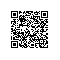# 利用抽象、多态实现无反射的绿色环保ORM框架

## ORM中反射的目的是什么？

ORM框架的种类形态各异，不同的公司不同的ORM实现。其实目的是为了能有一套属于自己公司的开发框架，这不是技术所定而是公司高层领导所要求的。(我们没有说话的权利，为了保住饭碗，我们只能听从指挥)

ORM是实体与表的一种映射关系，逐渐被发展为一种复杂的技术实现模型。

[Serializable()]

[HZ.Table(TableName = "member")]

public class Member{}

ORM中将实体的属性映射成数据库中表的字段，一般通过两种方式来表达这中关系。

[HZ.Column(PrimaryKey = true,ColumnName=”MemberId”)]

public string MemberCode { get; set; }

public string MemberId { get; set; }1. using System;
2. using System.Collections.Generic;
3. using System.Text;
4. using System.Collections;
5.
6. namespace Glory.Net.ORM
7. {
8.     public abstract class BaseEntityObject : DictionaryBase
9.     {
10.         /// <summary>
11.         /// 实体对象对应数据库中的表名
12.         /// </summary>
13.         private string _tablename = string.Empty;
14.         /// <summary>
15.         /// 受保护字典:实体类中对应数据库表中主键的属性和属性类型
16.         /// </summary>
17.         protected Dictionary<stringstring> _primarydictionary = new Dictionary<stringstring>();
18.         /// <summary>
19.         /// 用于实体子类设置当前子类对应数据库中的表名
20.         /// </summary>
21.         protected string TableName
22.         {
23.             get { return _tablename; }
24.             set { _tablename = value; }
25.         }
26.         /// <summary>
27.         /// 客户代码获取当前实例对应ORM中的表名
28.         /// </summary>
29.         public string GetTableName
30.         {
31.             get { return _tablename; }
32.         }
33.         /// <summary>
34.         /// 用于实体子类设置当前实例的属性值
35.         /// </summary>
36.         /// <param name="key"></param>
37.         /// <returns></returns>
38.         protected object this[string key]
39.         {
40.             get { return this.Dictionary[key]; }
41.             set { this.Dictionary[key] = value; }
42.         }
43.         /// <summary>
44.         /// 设置实例的属性值
45.         /// </summary>
46.         /// <param name="key">属性名称</param>
47.         /// <param name="value">属性值</param>
48.         public void SetEntityVlues(string key, object value)
49.         {
50.             if (this.Dictionary.Contains(key))
51.             {
52.                 this.Dictionary[key] = value;
53.             }
54.         }
55.         /// <summary>
56.         /// 获取实例的属性键值队
57.         /// </summary>
58.         /// <returns></returns>
59.         public IDictionary GetEntityValue
60.         {
61.             get{return (IDictionary)this.Dictionary;}
62.         }
63.         /// <summary>
64.         /// 获取实例的主键信息
65.         /// </summary>
66.         public IDictionary GetEntityPrimary
67.         {
68.             get { return (IDictionary)_primarydictionary; }
69.         }
71.     }
72. }

1. public class Tb_Car : VluesInitTb_Car
2.    {
3.        /// <summary>
4.        /// 唯一主键
5.        /// </summary>
6.        public string CID
7.        {
8.            get { return this["CID"as string; }
9.            set { this["CID"] = value; }
10.        }
11.        /// <summary>
12.        /// 车牌号
13.        /// </summary>
14.        public string CarBanrdCode
15.        {
16.            get { return this["CarBanrdCode"as string; }
17.            set { this["CarBanrdCode"] = value; }
18.        }
19.        /// <summary>
20.        ///
21.        /// </summary>
22.        public string CarCode
23.        {
24.            get { return this["CarCode"as string; }
25.            set { this["CarCode"] = value; }
26.        }
27.        /// <summary>
28.        ///
29.        /// </summary>
30.        public string DriverName
31.        {
32.            get { return this["DriverName"as string; }
33.            set { this["DriverName"] = value; }
34.        }
35.        /// <summary>
36.        /// 联系电话
37.        /// </summary>
38.        public string Mobile
39.        {
40.            get { return this["Mobile"as string; }
41.            set { this["Mobile"] = value; }
42.        }
43.        /// <summary>
44.        /// 车型
45.        /// </summary>
46.        public string CarType
47.        {
48.            get { return this["CarType"as string; }
49.            set { this["CarType"] = value; }
50.        }
51.        /// <summary>
52.        /// 购车日期
53.        /// </summary>
55.        {
56.            get { return this["BuyDateTime"as DateTime?; }
57.            set { this["BuyDateTime"] = value; }
58.        }
59.        /// <summary>
60.        /// 所属中心编号。外键
61.        /// </summary>
62.        public string AttachCenter
63.        {
64.            get { return this["AttachCenter"as string; }
65.            set { this["AttachCenter"] = value; }
66.        }
67.        /// <summary>
68.        /// 所属区部编号。外键
69.        /// </summary>
70.        public string AttachSection
71.        {
72.            get { return this["AttachSection"as string; }
73.            set { this["AttachSection"] = value; }
74.        }
75.        /// <summary>
76.        /// 所属站点编号。外键
77.        /// </summary>
78.        public string AttachStop
79.        {
80.            get { return this["AttachStop"as string; }
81.            set { this["AttachStop"] = value; }
82.        }
83.    }

1. [Serializable()]
2.    public class VluesInitTb_Car : BaseEntityObject
3.    {
4.        public VluesInitTb_Car()
5.        {
6.            this.TableName = "Tb_Car";
7.            /// <summary>
8.            /// 唯一主键
9.            /// </summary>
11.            /// <summary>
12.            /// 车牌号
13.            /// </summary>
15.            /// <summary>
16.            ///
17.            /// </summary>
19.            /// <summary>
20.            ///
21.            /// </summary>
23.            /// <summary>
24.            /// 联系电话
25.            /// </summary>
27.            /// <summary>
28.            /// 车型
29.            /// </summary>
31.            /// <summary>
32.            /// 购车日期
33.            /// </summary>
35.            /// <summary>
36.            /// 所属中心编号。外键
37.            /// </summary>
39.            /// <summary>
40.            /// 所属区部编号。外键
41.            /// </summary>
43.            /// <summary>
44.            /// 所属站点编号。外键
45.            /// </summary>
48.        }
49.
50.        /// <summary>
51.        /// 实体类 重写实体基类添加主键信息方法，主键数据类型首字母要大写
52.        /// </summary>
54.        {
56.        }
57.    }

ORM通过实例化一个对象的实例然后将其缓存起来，作为后续使用。而不需要频繁的实例化中间对象带来的性能问题。

本文转自 王清培 51CTO博客，原文链接：http://blog.51cto.com/wangqingpei557/855135，如需转载请自行联系原作者使用钉钉扫一扫加入圈子
+ 订阅